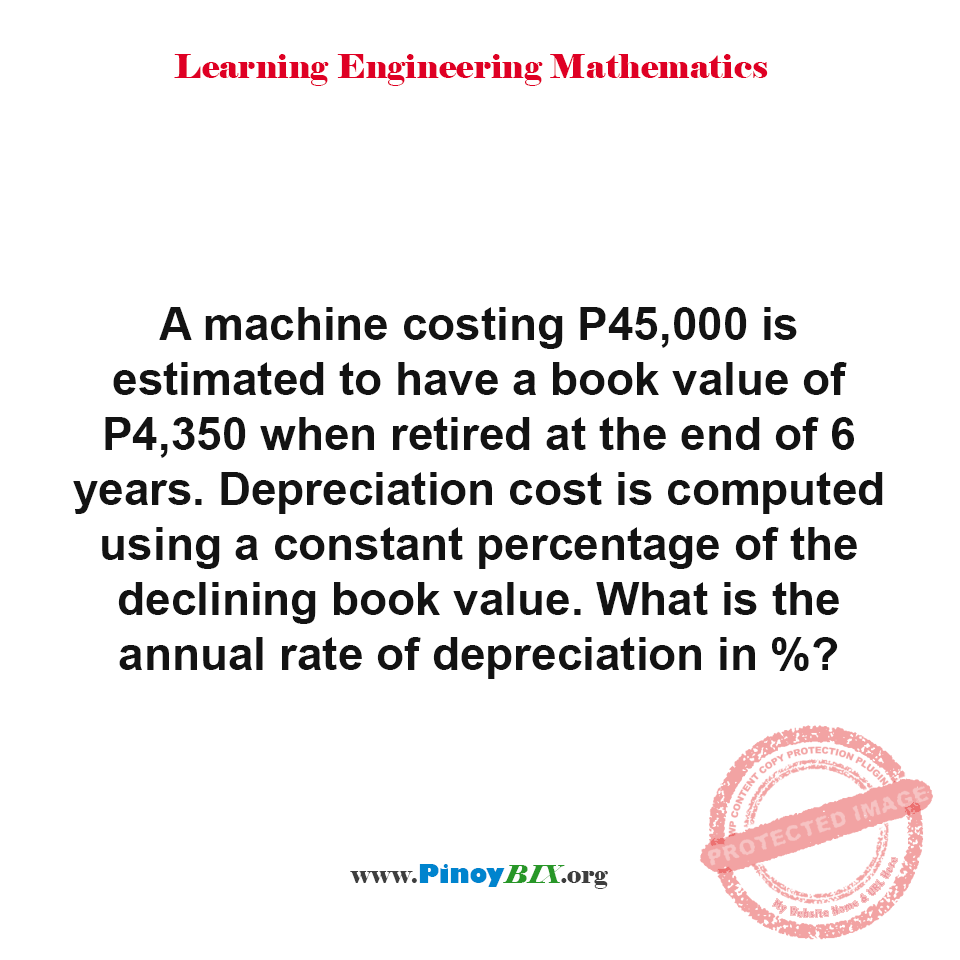# Solution: Find the annual rate of depreciation of the machine in percentage

(Last Updated On: January 9, 2020)#### Problem Statement: CE Board May 1996

A machine costing P45,000 is estimated to have a book value of P4,350 when retired at the end of 6 years. Depreciation cost is computed using a constant percentage of the declining book value. What is the annual rate of depreciation in %?

• A. 32.50%
• B. 32.25%
• C. 32.00%
• D. 32.75%

The annual rate of depreciation of the machine is 32.25%.

Solution:

### Latest Problem Solving in Engineering Economics (Annuity, Depreciation, Bonds, Breakeven, etc.)

More Questions in: Engineering Economics (Annuity, Depreciation, Bonds, Breakeven, etc.)

#### Online Questions and Answers in Engineering Economics (Annuity, Depreciation, Bonds, Breakeven, etc.)

PinoyBIX Engineering. © 2014-2021 All Rights Reserved | How to Donate? |